# Utilities - Standalone Statistics test

SUMO performs several different statistical test with alld data from a data mtrix.

For convenience, some of these tests may be performed interactively on sigular data sets.

## Kolmogorov-Smirnov test

Wikipedia explains:
"... the Kolmogorov-Smirnov test (K-S test or KS test) is a nonparametric test of the equality of continuous, one-dimensional probability distributions that can be used to compare a sample with a reference probability distribution (one-sample K-S test), or to compare two samples (two-sample K-S test). ...
... The two-sample K-S test is one of the most useful and general nonparametric methods for comparing two samples, as it is sensitive to differences in both location and shape of the empirical cumulative distribution functions of the two samples. ..."

Use this utilitiy to test equality of your data.

In SUMO select Main menu | Utilities | Statistic tests | Kolmogorov-Smirnov test

The KS-test dialog opens up: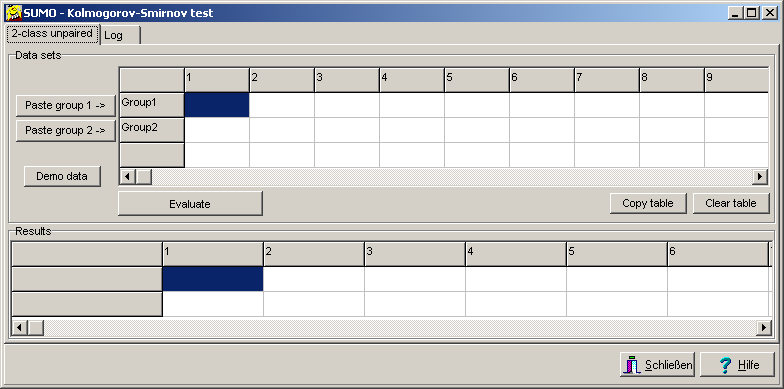Fill in data values for group1 and group2.
Or copy Tab/NewLine/space separated values into ClipBoard.
Click Paste group1/2 button to paste clipboard content into the respective data grid row.
(SUMO tries to convert German "," nto international "." decimal divider)

Click the Evaluate butto to perform the KS-test.

Results are shown in the Resutls table: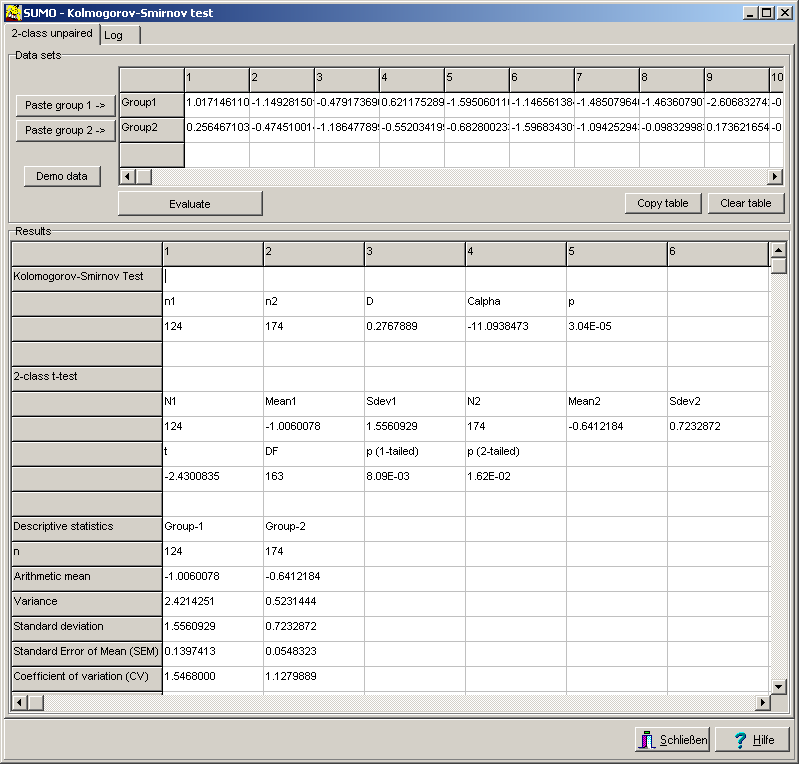The Result table indicates:

 Top: Results from KS-test Middle: For comparison - results from t-test Bottom: Some descriptive statistics

• density function of pasted data
• Empirical Distribution Function (EDF) of pasted data (~cumulative density function)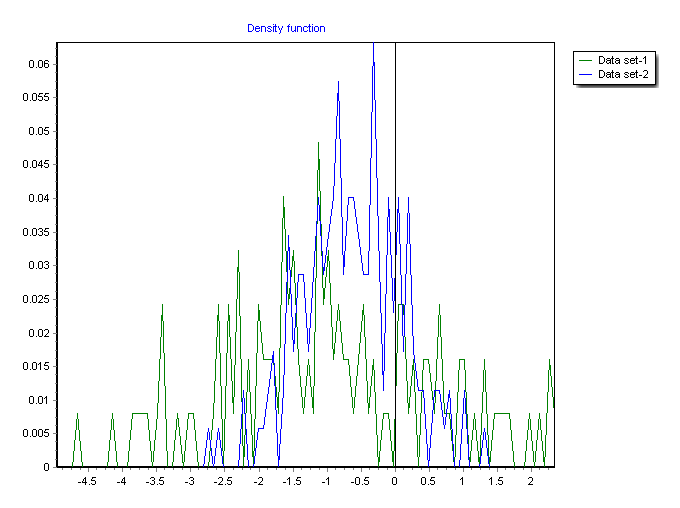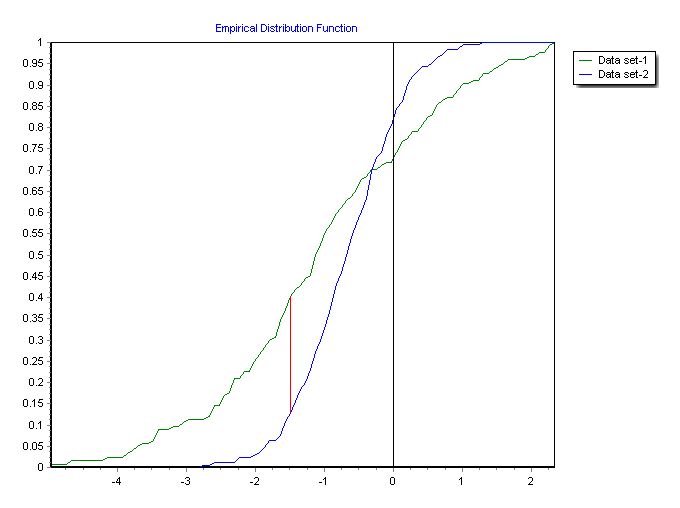To experiment with KS-test, try Demo data.

 2 identical gaussians Two different randomly samples Gaussian distributed data sets. Both with Mean=0, SDev=1 2 gaussians different mean Two different randomly samples Gaussian distributed data sets. Both with different Mean, similar SDev 2 gaussians, different SDev Two different randomly samples Gaussian distributed data sets. Both with similar Mean, different SDev 2 different gaussians Two different randomly samples Gaussian distributed data sets. Both with different Mean and SDev Gaussian <=> Random A Gaussian distributed data sets versus a random dataset. Random <=> Random Two different random dataset.

For more details about KS-test see Non parametric tests | Kolmogorov-Smirnov test.

## 2x2 Cross tables / Contingency tables

A 2x2 Contingency table shows the frequency of the respective two states in two varaibals.

A simple example:
Analyse the handedness of members ina group of individual depending ongender:
Handedness
Left-handedright-handedSum
GenderFemale44448
Male43952
Sume8713100

Very often 2x2 tables are used to compare predicted paramters versius observed paramters.
Here thea are called Confusion matrix.
Predicted
PosiivteNegative
ObservedPositiveTrue PositiveFalse Negative
NegativeFalse PositiveTrue Negative

With the values TP,FP,FN,TN we can derive various values to indicate association betweeen the two variables (Predicted/Observed):

 TP True positives (Hits) FP False positives (Class 1 error) FN False negatives (Class 2 error) TN True negatives (Correct rejection) SPC Specificity = TN / (FP+TN) = True Negative Rate (TNR) = Selectivity SEN Sensitivity = TP / (TP+FN) = True Positive Rate (TPR) = Recall = Hit rate PPV Positive Predictive Value = TP / (TP+FP) = Precision NPV Negative Predictive Value = TN / (TN+FN) PRE Prevalence = (TP+FN)/(TP+FP+FN+TN) ACC Accuracy = (TP+TN)/(TP+FP+FN+TN) = Random precision BA Balance Accuracy = (TPR+TNR)/2 FPR False Positive Rate = FP / (FP+TN) = fall out FNR False Negative Rate = FN ( FN+TP) = Miss rate Gain PPV / ACC MCC Matthews Correlation Coefficient = ((TP*TN)-(FP*FN)) / sqrt((TP+FP)*(FN+TN)*(TP+FN)*(FP*TN)) = phi-Coefficient FDR False Dicovery Rate = FP / (FP+TP) FOR False Omission Rate = FN / (FN+TN) LR+ Positive Likelihood ratio = TPR/FPR = Senistivity/FPR LR- Negative Likelihood ratio = FNR/TNR = FNR/Specificity FS F-Score = 2*TP / (TP+FP+TP+FN) = F1-Score or F Measure RR Relative Risk = TP/(TP+FP) / FN/(FN+TN) DOP difference between disproportion = | TP/(TP+FP) - FN/(FN+FP) PT Prevalence Threshold = sqrt(FPR) / (sqrt(TPR)+sqrt(FPR)) TS Threat Score = Critical Succes Index (CSI = TP / (TP+FN+FP) FM Fowlkes-Mallows Index = sqrt(PPV*TPR) BM Bookmarker Informdness = Informdness = TPR + TNR -1 MK MArkness = DeltaP = PPS+NPV-1 Odds ratio (TP/FP) / (FN/TN)' pDOF Significance by Pearson''s Goodness-of-Fit Test' pFET Significance by Fisher''s Exact test Yule's Q Yule coefficient of association = (TP-TN) / (TP+TN) YuleS's Y coefficient of colligation = = 1 - sqrt( 1-sqr(1-YulesQ)) / YulesQ

Similarity measures< - as used in clustering of binary verctors - can be computed:

Simple Matching
Russel-Rao
Tanimoto
Kulczynski
Braun
Hamann<
Cohen's Kappa
Bandigwala
Ochiai
Phi
Sneath
Simpson
Yule
Accuracy
F1-Score

From SUMO slect

MAin menu | Utilities | Statistic tests | 2x2 cross table

A new Winwow opens up: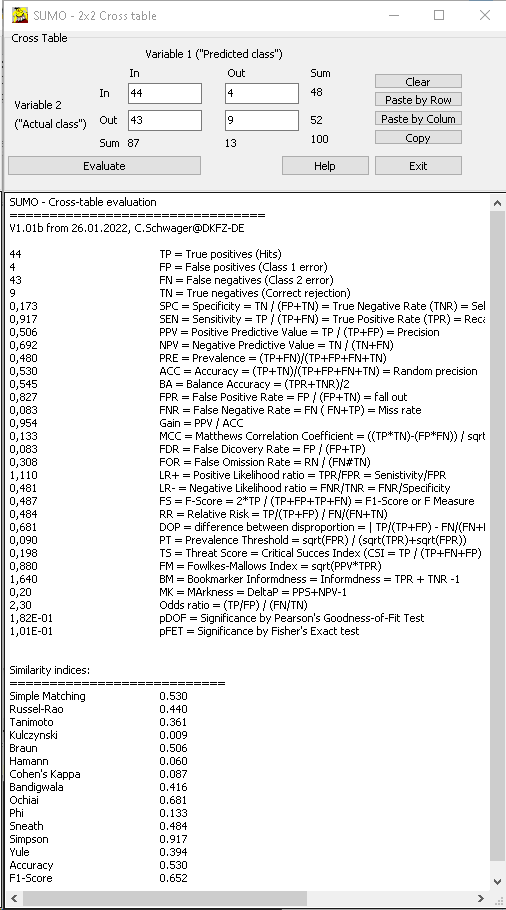Fill in the values und press Evaluate button to compute the association values.

## Correlation coefficient r => p-value

What is the probability that a given correlaton value (r) measured with a given number of samples (n) is just random (p~1) or very unexpected (p<<0) ?

In a first step we can compute a t-value: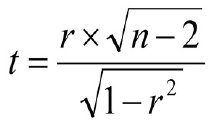r should be in the range: -1 < r < 1, n > 2

(adopted from: Miles and Banyard's (2007), Understanding and Using Statistics in Psychology --- A Practical Introduction)

From t-distribution we can find the corresponding p-value.

In SUMO select Main menu | Utilities | Statistic tests | Correlation coefficient => p-value

Enter your data (r and n).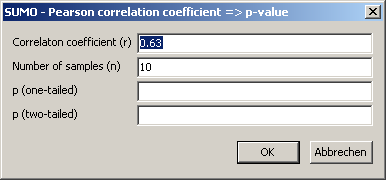Click OK-button to compute p:Edit r / n and recompute p, or Cancel this utility.

## Probability => Correlation coefficient r

What correlation coefficent r do I need to yield a certain probability value p ?

In a first step, we can get the t-value from an inverse t-distrubtion for the desired p-value.

Applying and converting the above formula we can compute r: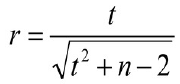In SUMO select Main menu | Utilities | Statistic tests | p-value => Correlation coefficient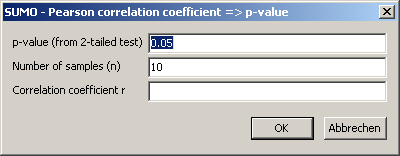Click OK-button to compute p: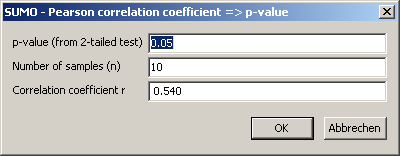Edit p / n and recompute r, or Cancel this utility.

## Mantel test - Test for similarity of two matrices

Wikipedia explains:
The Mantel test, named after Nathan Mantel, is a statistical test of the correlation between two matrices. The matrices must be of the same dimension; in most applications, they are matrices of interrelations between the same vectors of objects.
Originally the Mantel test was introduced to compare distance matrices: square matrices with identical dimensions and positive data values.
But the tst may be also performed with not squared matrices.

The two matrices must have:
• Same dimensions (i.e. same number of rows/columns).
In case the two matrices have different dimensions, SUMO truncates the larger one to the dimension of the smaller one.
• Positive data values
negative data values are processed too, but the test result may be meaningless
• All data cells should contain numbers.
SUMO converts non numeric data or empty data cells to ZERO
• Numbers hould be supplied in international format (decimal-point as divider)
SUMO tries to convert german format (decimal-comma)

Mantel's test statistic is comupted with a basic cross product formula: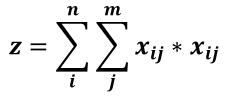With x,y two (square) non-negative matrices with n, m number of rows/columns.

A normalized correlation value is computed: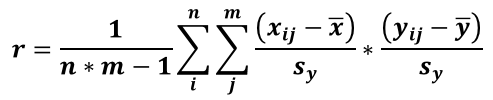with ‾x, ‾y = average from matrix x and y respectively.
sx, sy, = standard deviation from matrix x and y respectively

r ranges from -1 ... 1-
• r=1 : highest similarity, matrices are identical
• r~0 : just random values, no similarity
• r=-1 : matrices are contradictory

A significance value for similarity is computed based on a permutation scheme wher rows and columns of (one) matrix are radomly shuffeld.
For each of the np permutation an r' is computed
The number m of permutations where r' < r is counted and onverted into a p-value:
p = (m+1) / np

To extract a trustful p-value, the number of permutations should be adopted to the critical p-value:
pnp
0.051000
0.015000
0.001   50000
......

With SUMO select:

Main menu | Utilities | Statistics | Mantel test

In the parameter dialog select/specify:
• Data matrix files: either type the names, separated by semicolon
click the  ...   button to open a file selection box
drag files from file explorer into the data-matrix field
• Header rows/columns: define number of such rows/coloms, containing description/annotions not useful for computation
Header rows/colums MUST be identical in the matrices
• Number of permutation cycle for computation of p-value